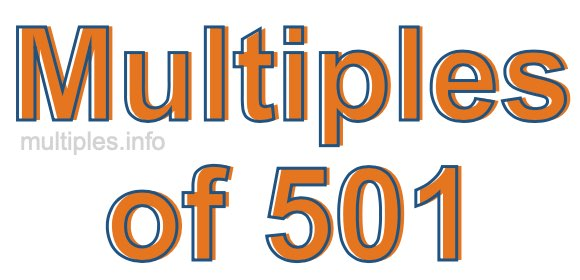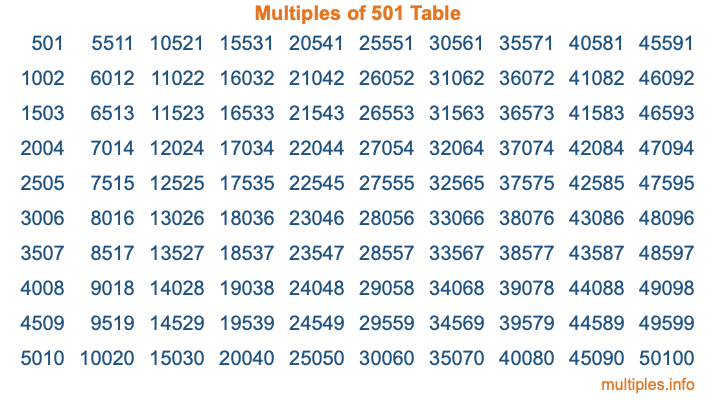Multiples of 501Welcome to the Multiples of 501 page. Here we will first teach you everything you will ever need to know about the multiples of 501, and then give you a study guide summary of everything we taught you to make sure you remember it all. Use this page to look up facts and learn information about the multiples of 501. This page will make you a multiples of five hundred one expert!

Definition of Multiples of 501
Multiples of 501 are all the numbers that when divided by 501 equal an integer. Each of the multiples of 501 are called a multiple. A multiple of 501 is created by multiplying 501 by an integer.

Therefore, to create a list of multiples of 501, you start with 1 multiplied by 501, then 2 multiplied by 501, then 3 multiplied by 501, and so on for as long as you want. Thus, the list of the first five multiples of 501 is 501, 1002, 1503, 2004, and 2505. To see a larger list of multiples of 501, see the printable image of Multiples of 501 further down on this page. We also have a category where you can choose any nth multiple of 501.

Multiples of 501 Checker
The Multiples of 501 Checker below checks to see if any number of your choice is a multiple of 501. In other words, it checks to see if there is any number (integer) that when multiplied by 501 will equal your number. To do that, we divide your number by 501. If the the quotient is an integer, then your number is a multiple of 501.

Is  a multiple of 501?

Least Common Multiple of 501 and ...
A Least Common Multiple (LCM) is the lowest multiple that two or more numbers have in common. This is also called the smallest common multiple or lowest common multiple and is useful to know when you are adding our subtracting fractions. Enter one or more numbers below (501 is already entered) to find the LCM.

Check out our LCM Calculator if you need more details about the Least Common Multiple or if you need the LCM for different numbers for adding and subtraction fractions.

nth Multiple of 501
As we stated above, 501 is the first multiple of 501, 1002 is the second multiple of 501, 1503 is the third multiple of 501, and so on. Enter a number below to find the nth multiple of 501.

th multiple of 501

Multiples of 501 vs Factors of 501
501 is a multiple of 501 and a factor of 501, but that is where the similarities end. All postive multiples of 501 are 501 or greater than 501. All positive factors of 501 are 501 or less than 501.

Below is the beginning list of multiples of 501 and the factors of 501 so you can compare:

Multiples of 501: 501, 1002, 1503, 2004, 2505, etc.

Factors of 501: 1, 3, 167, 501

As you can see, the multiples of 501 are all the numbers that you can divide by 501 to get a whole number. The factors of 501, on the other hand, are all the whole numbers that you can multiply by another whole number to get 501.

It's also interesting to note that if a number (x) is a factor of 501, then 501 will also be a multiple of that number (x).

Multiples of 501 vs Divisors of 501
The divisors of 501 are all the integers that 501 can be divided by evenly. Below is a list of the divisors of 501.

Divisors of 501: 1, 3, 167, 501

The interesting thing to note here is that if you take any multiple of 501 and divide it by a divisor of 501, you will see that the quotient is an integer.

Multiples of 501 Table
Below is an image of the first 100 multiples of 501 in a table. The table is in chronological order, column by column. The first column has the first ten multiples of 501, the second column has the next ten multiples of 501, and so on.The Multiples of 501 Table is also referred to as the 501 Times Table or Times Table of 501. You are welcome to print out our table for your studies.

Negative Multiples of 501
Although not often discussed or needed in math, it is worth mentioning that you can make a list of negative multiples of 501 by multiplying 501 by -1, then by -2, then by -3, and so on, to get the following list of negative multiples of 501:

-501, -1002, -1503, -2004, -2505, etc.

Multiples of 501 Summary
Below is a summary of important Multiples of 501 facts that we have discussed on this page. To retain the knowledge on this page, we recommend that you read through the summary and explain to yourself or a study partner why they hold true.

There are an infinite number of multiples of 501.

A multiple of 501 divided by 501 will equal a whole number.

501 divided by a factor of 501 equals a divisor of 501.

The nth multiple of 501 is n times 501.

The largest factor of 501 is equal to the first positive multiple of 501.

501 is a multiple of every factor of 501.

501 is a multiple of 501.

A multiple of 501 divided by a divisor of 501 equals an integer.

501 divided by a divisor of 501 equals a factor of 501.

Any integer times 501 will equal a multiple of 501.

Multiples of a Number
Here you can get the multiples of another number, all with the same attention to detail as we did for multiples of 501 on this page.

Multiples of
Multiples of 502
Did you find our page about multiples of five hundred one educational? Do you want more knowledge? Check out the multiples of the next number on our list!